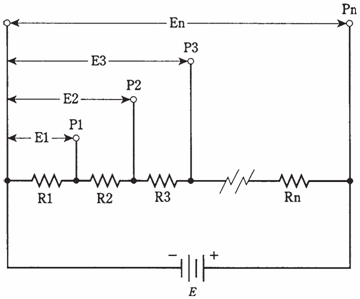## Voltage divider networks Assignment Help

Assignment Help: >> Electrical Engineering - Voltage divider networks

Voltage divider networks:

You can imagine, nightmarish series-parallel matrixes of resistors drawn all over whole sheets of paper by now, captioned with wicked queries: What is the current through the R135? But that stuff is best left to the professional engineers, and even they are not to come across it very often. Their job is to make things as efficient as possible. If an engineer is faced with such a scenario, the reaction will probably be, to think about how these problems can be simplified.

Resistances in the series produce ratios of voltages, and these ratios can be tailored to meet certain requirements.

When designing voltage divider networks, the resistance values should be very small, without causing too much current drain on the supply. In practice the values depend on nature of the circuit which is being designed. The reason for electing the smallest possible resistances is that, when the divider is used along with the circuit, you do not want that circuit to upset the operation of divider. The voltage divider "fixes" intermediate voltages best when resistance values are as small as current delivering capability of the power supply will allow.

Figure given below shows the principle of voltage division. The individual resistances are R1, R2, R3, ... Rn. The total resistance is R+R1+R2+R3...Rn. Then the supply voltage is E, and current in circuit can be given by I=  E/R. At the several points P1, P2, P3,, ... Pn, voltages will be E1, E2, E3, ..., En. The last voltage, En, is same as supply voltage, E. All the other voltages are much less than E, so E1<E2<E3<...  En =E.Figure--   General arrangement for voltage divider circuit.

The voltages at several points increase according to the sum total of resistances up to each point, in proportion to total resistance, multiplied by supply voltage. The voltage E1 is equal to E=R1/R. The voltage E2 is equal to E*(R1+R2)/RThe voltage E3 is E *(R1 +R2+R3)/RYou can continue the process to obtain voltages at points all the way up to E=E*(R1+R2+R3+...Rn)/R = E * R/R= E*1 = E.